# R S Aggarwal Solutions for Class 10 Maths Chapter 8 Circles Exercise 8B

R S Aggarwal Solutions for Class 10 Maths exercise 8B are available here. These Solutions are the best study material for the students appearing in the board exams.

Chapter 8 exercise 8B mainly deals with problems based on tangent theorems. Students can download R S Aggarwal Solutions for Maths chapter 8 to clear their concepts on circles.

## Download PDF of R S Aggarwal Solutions for Class 10 Maths Chapter 8 Circles Exercise 8B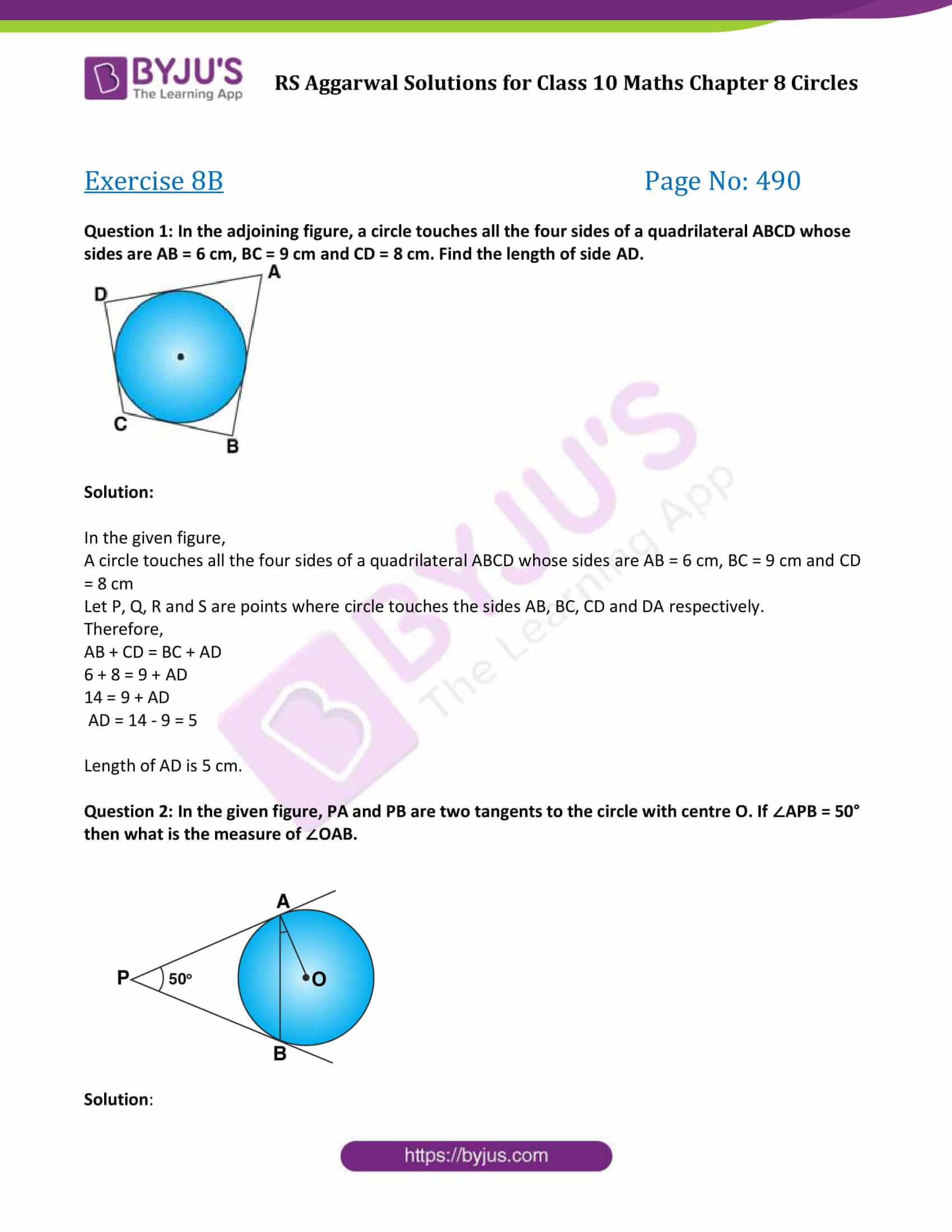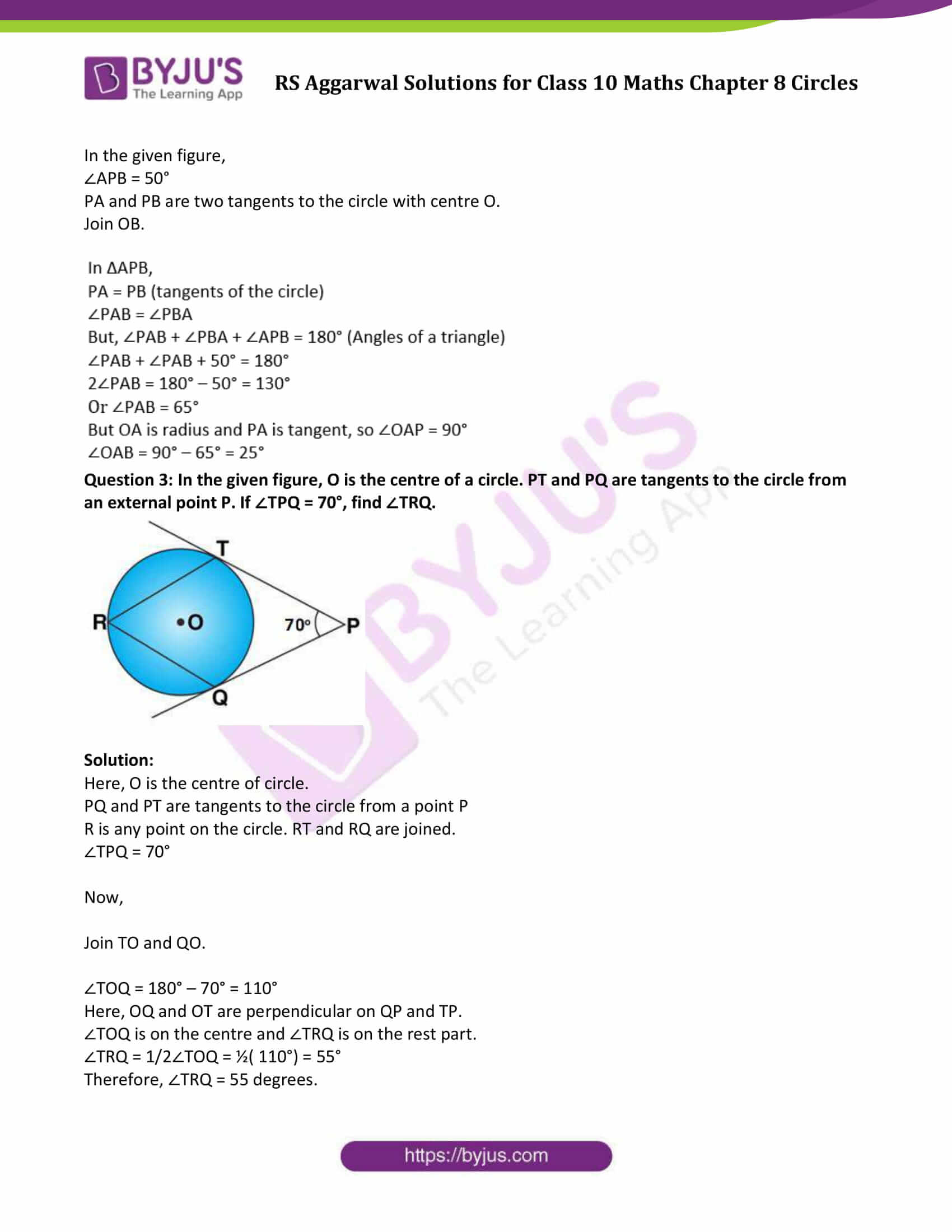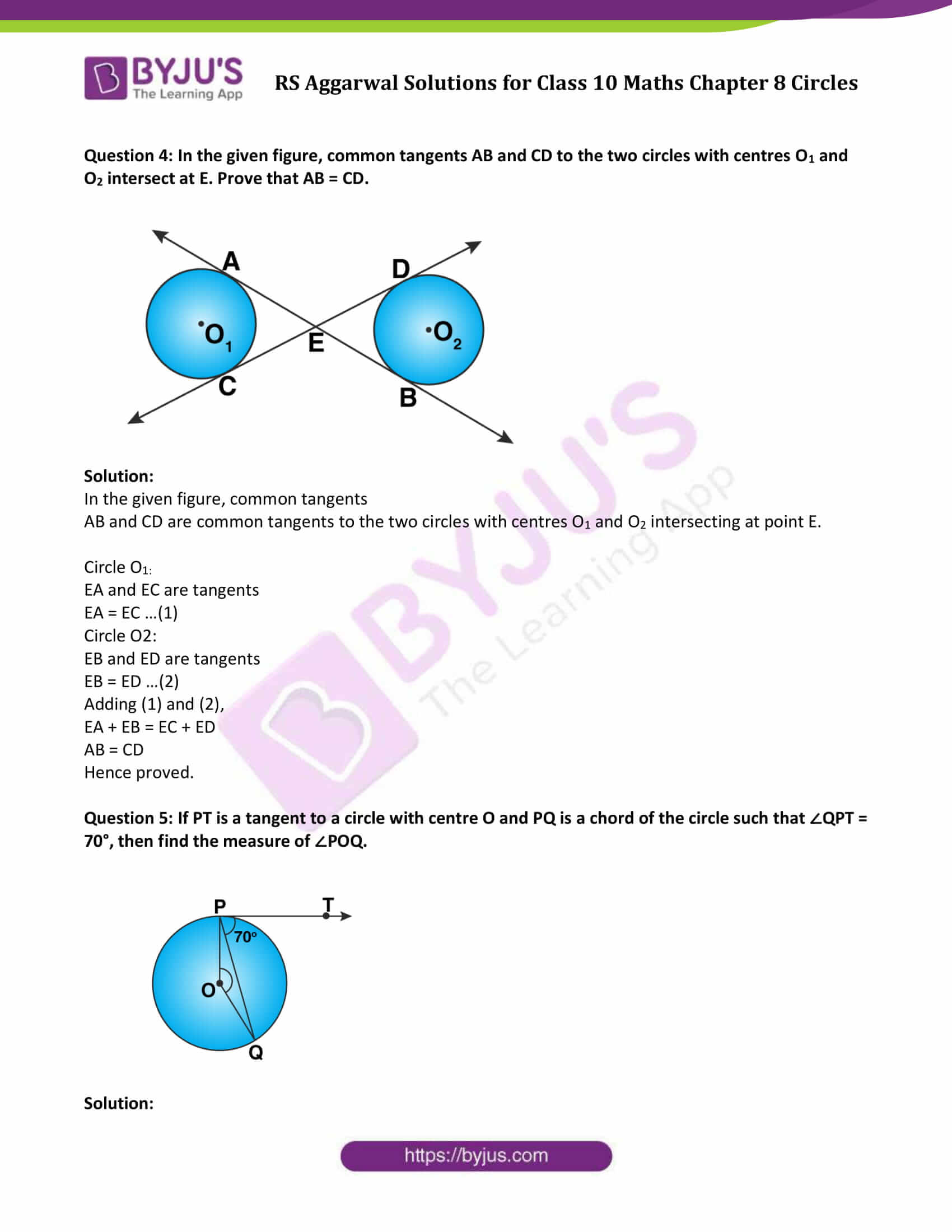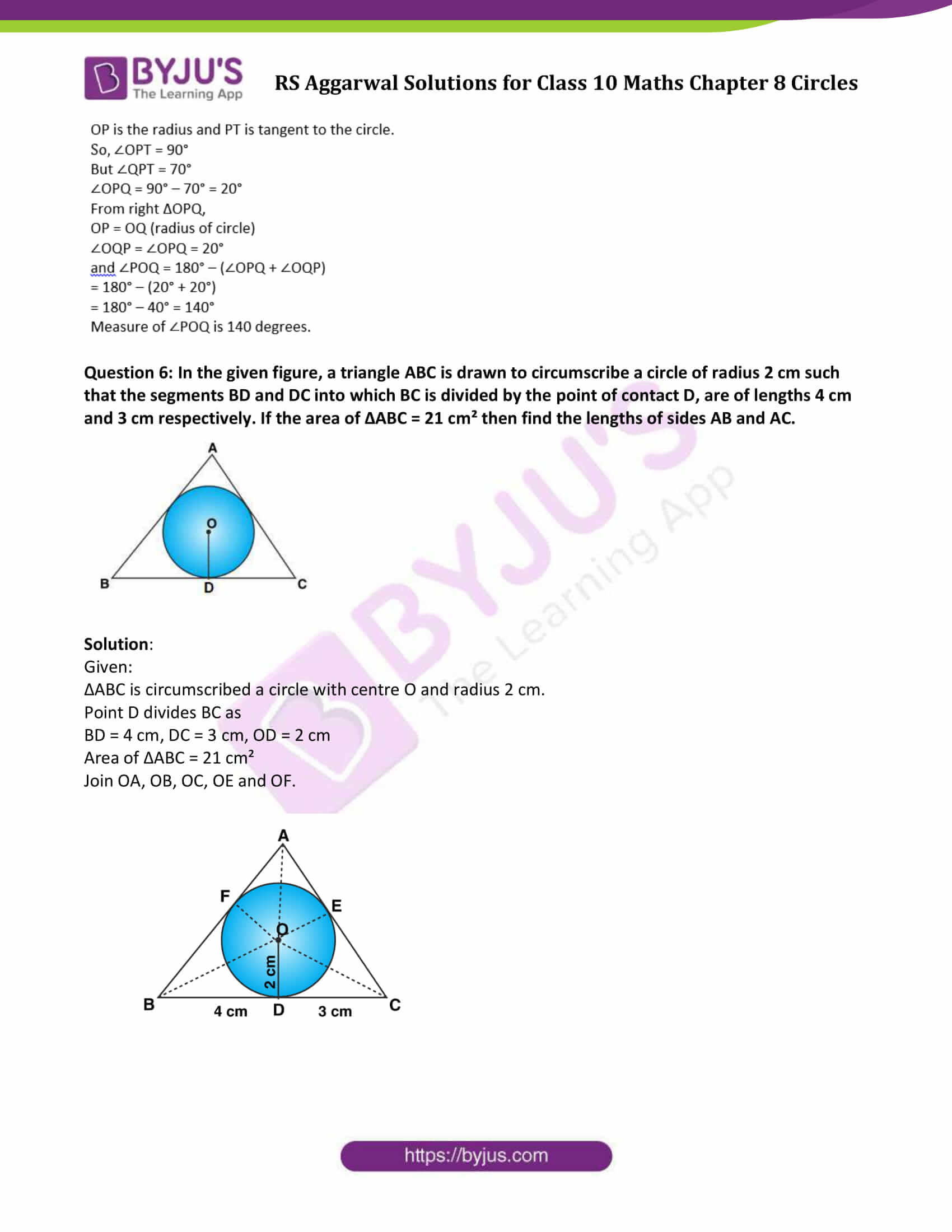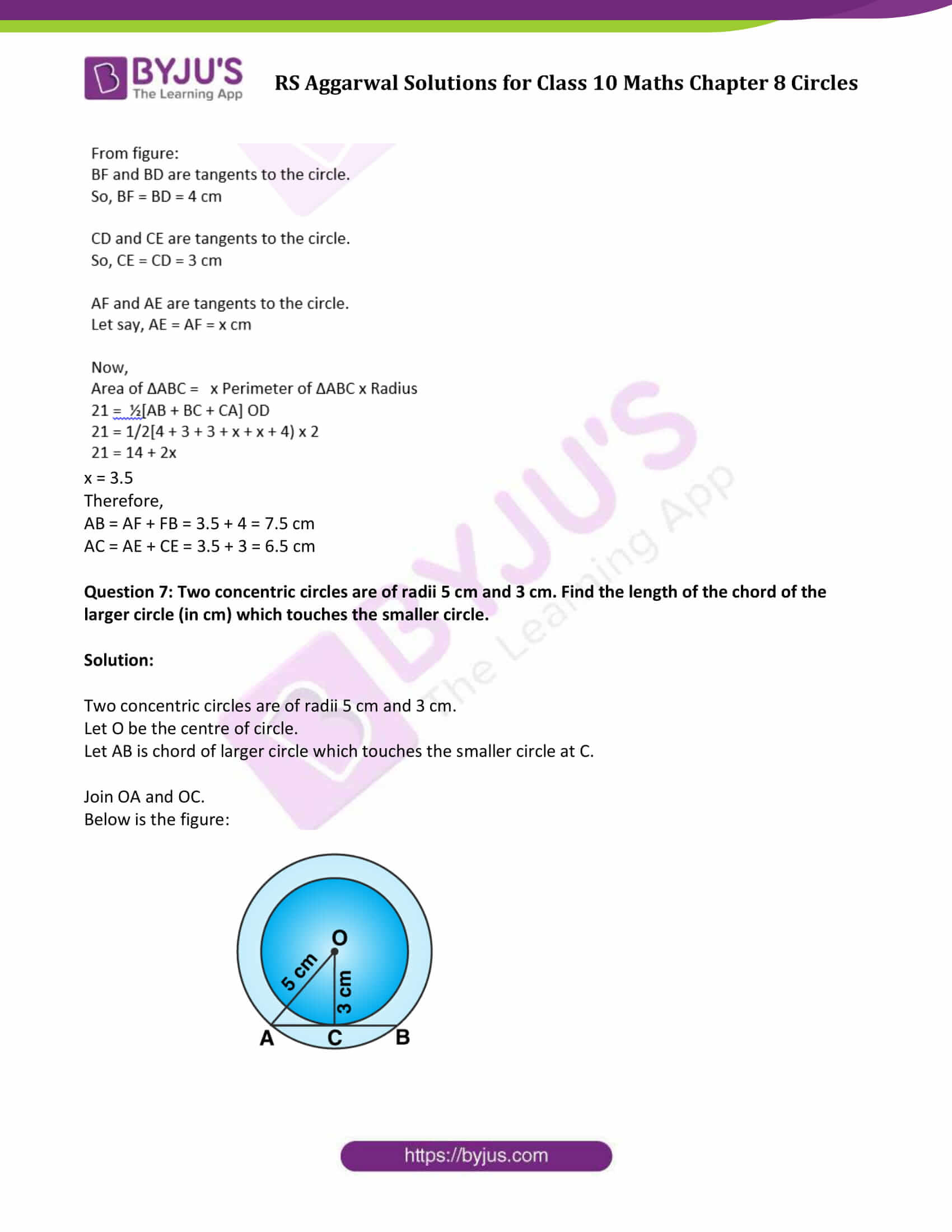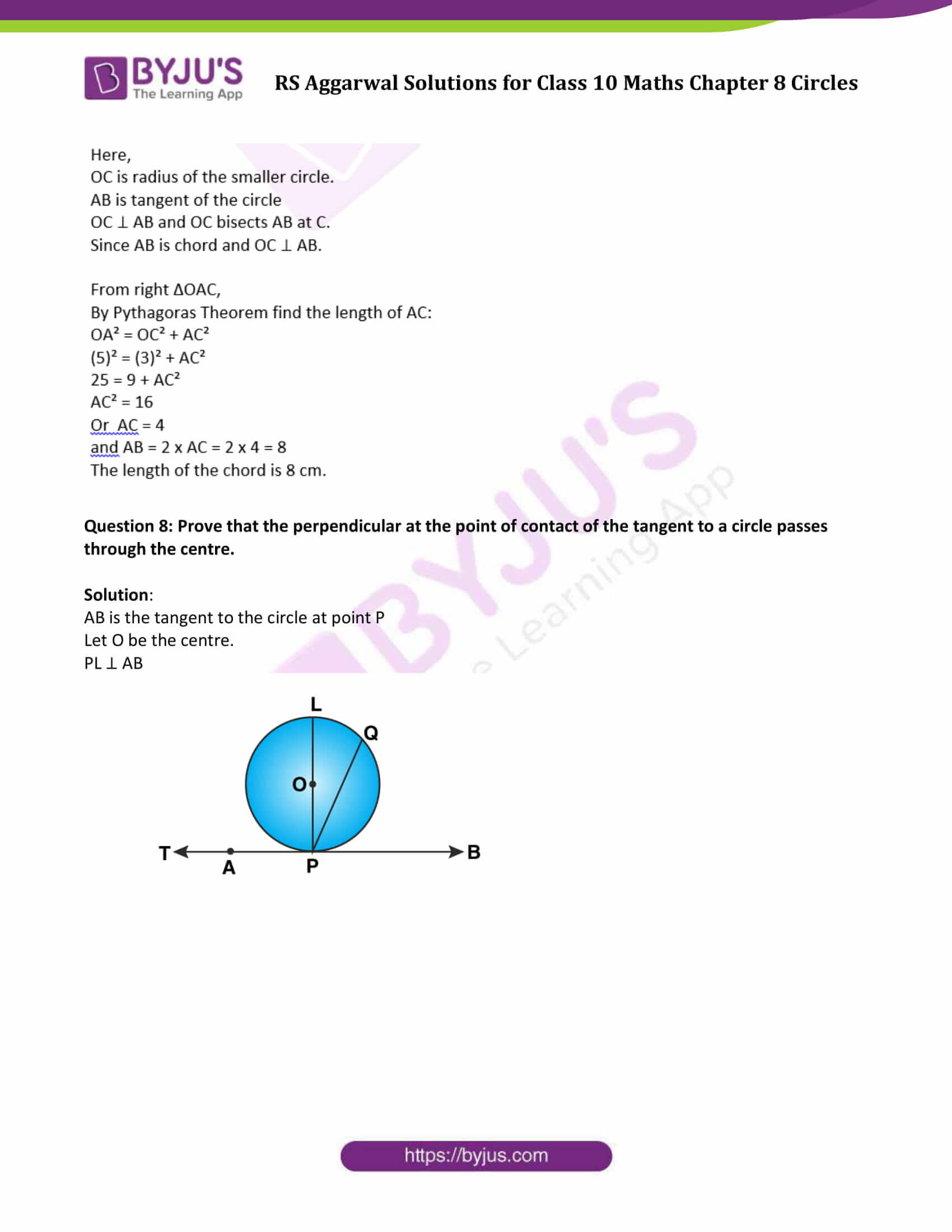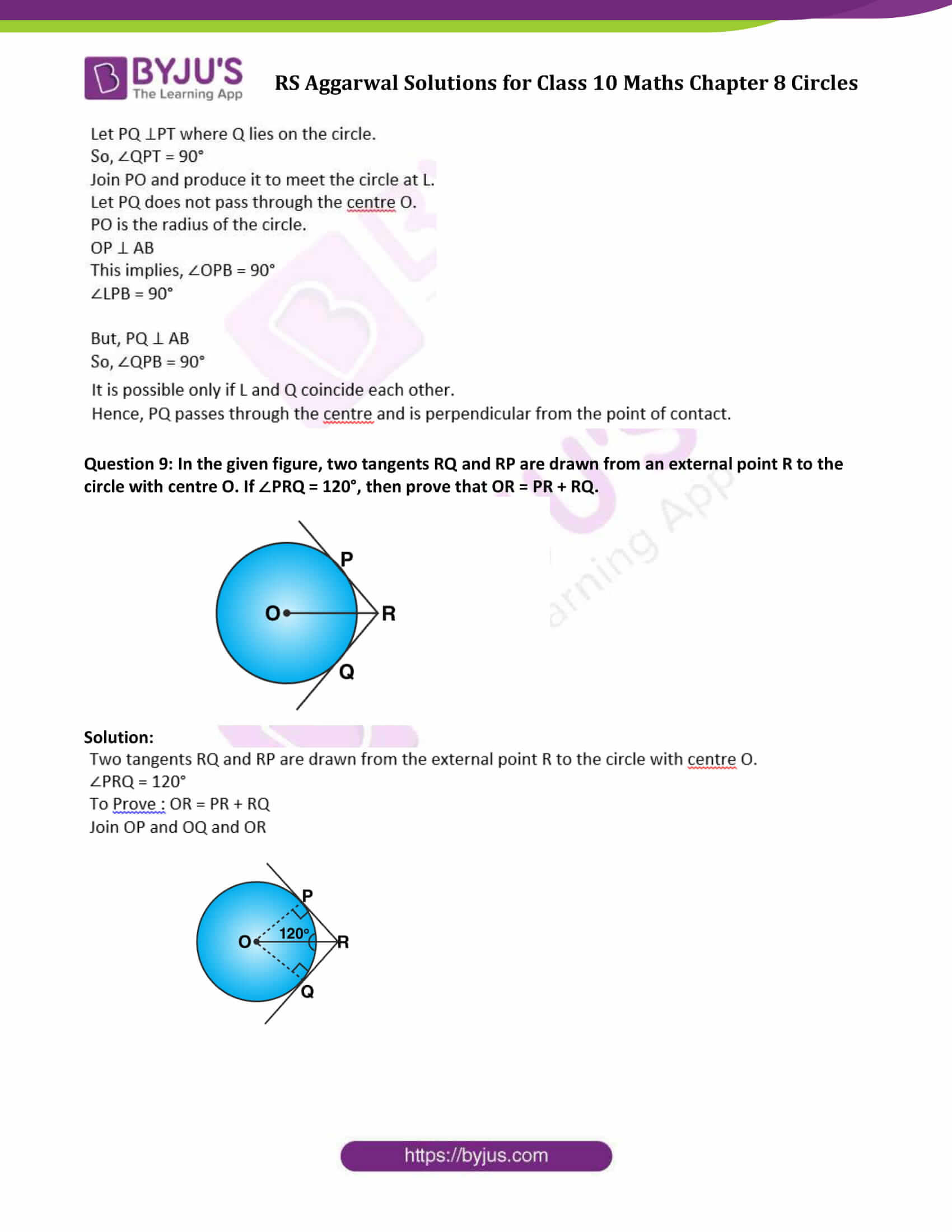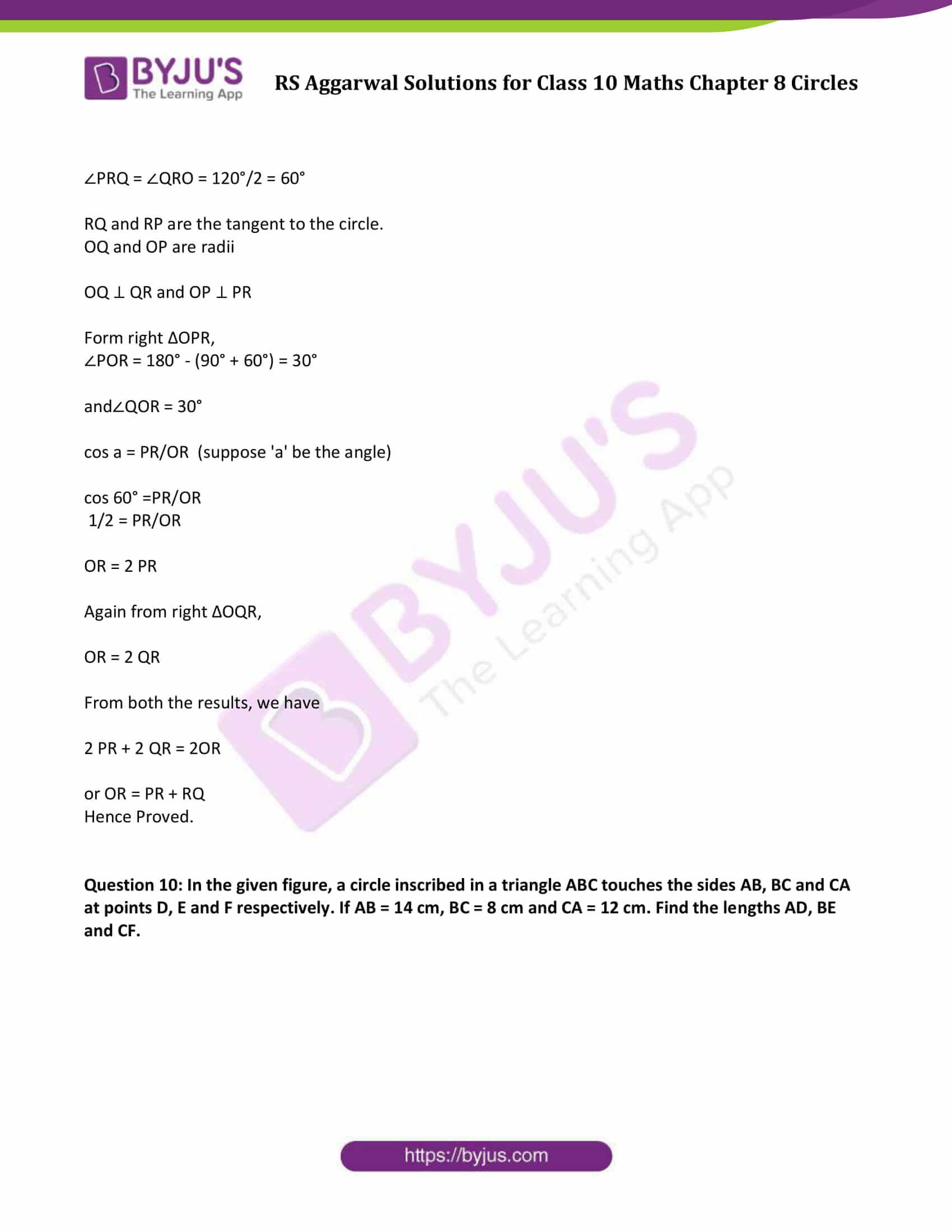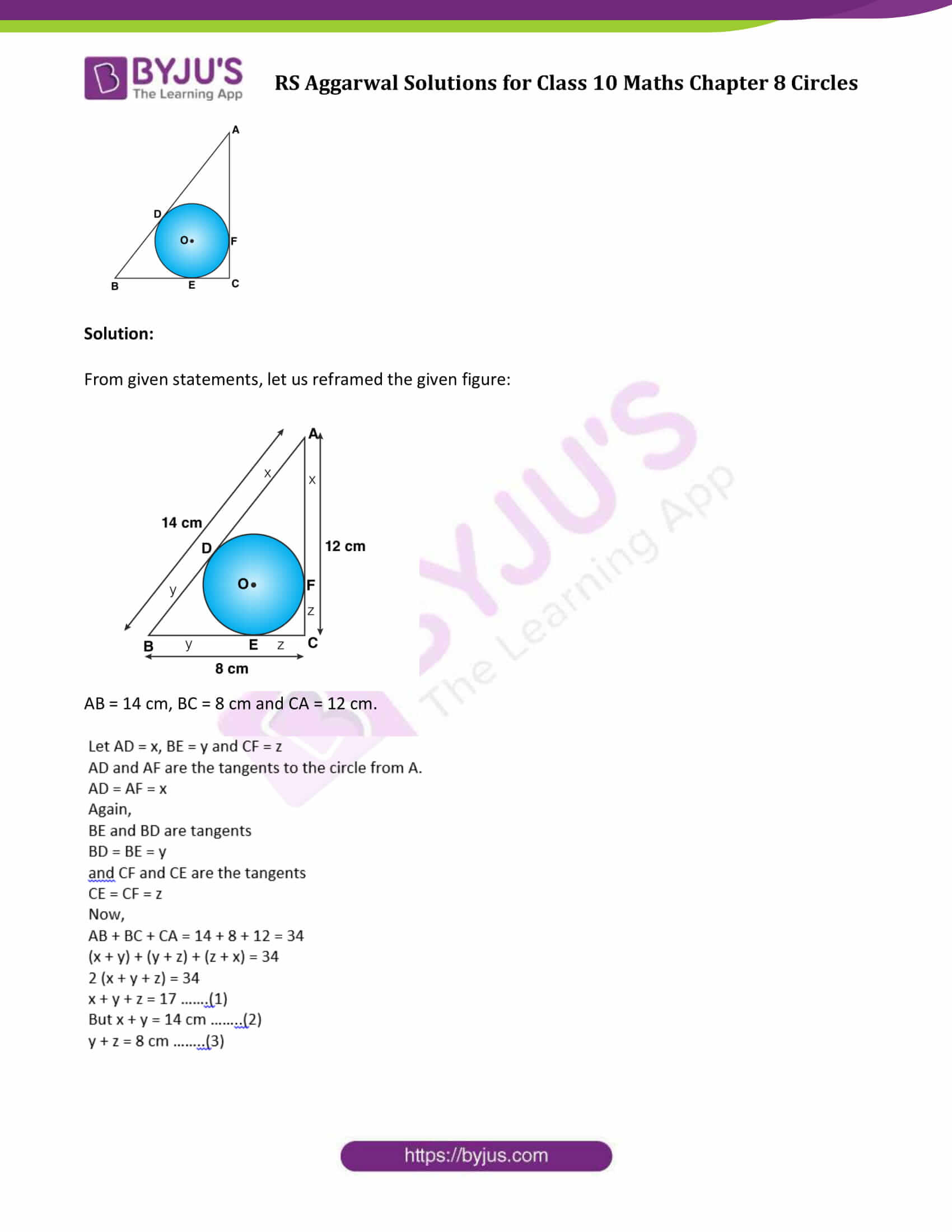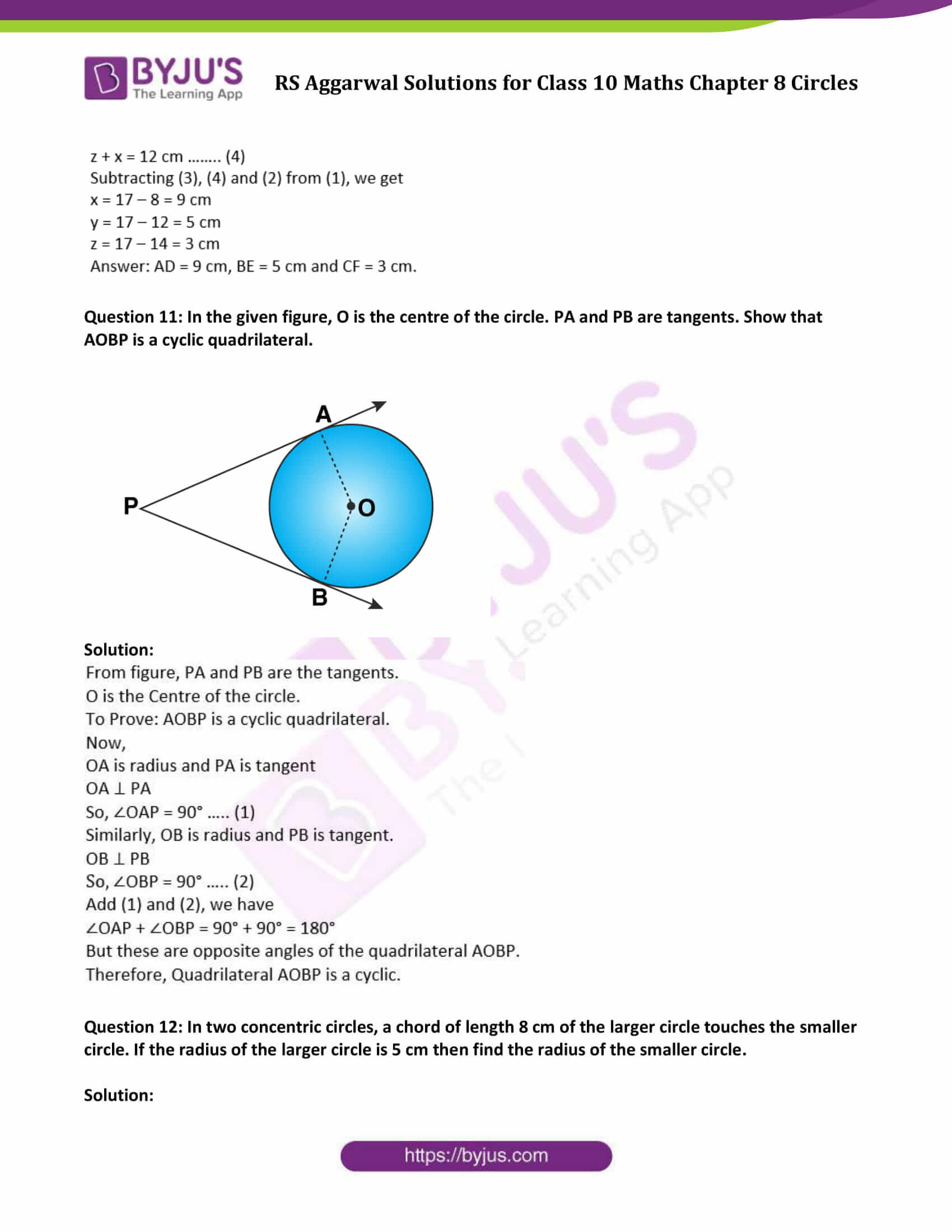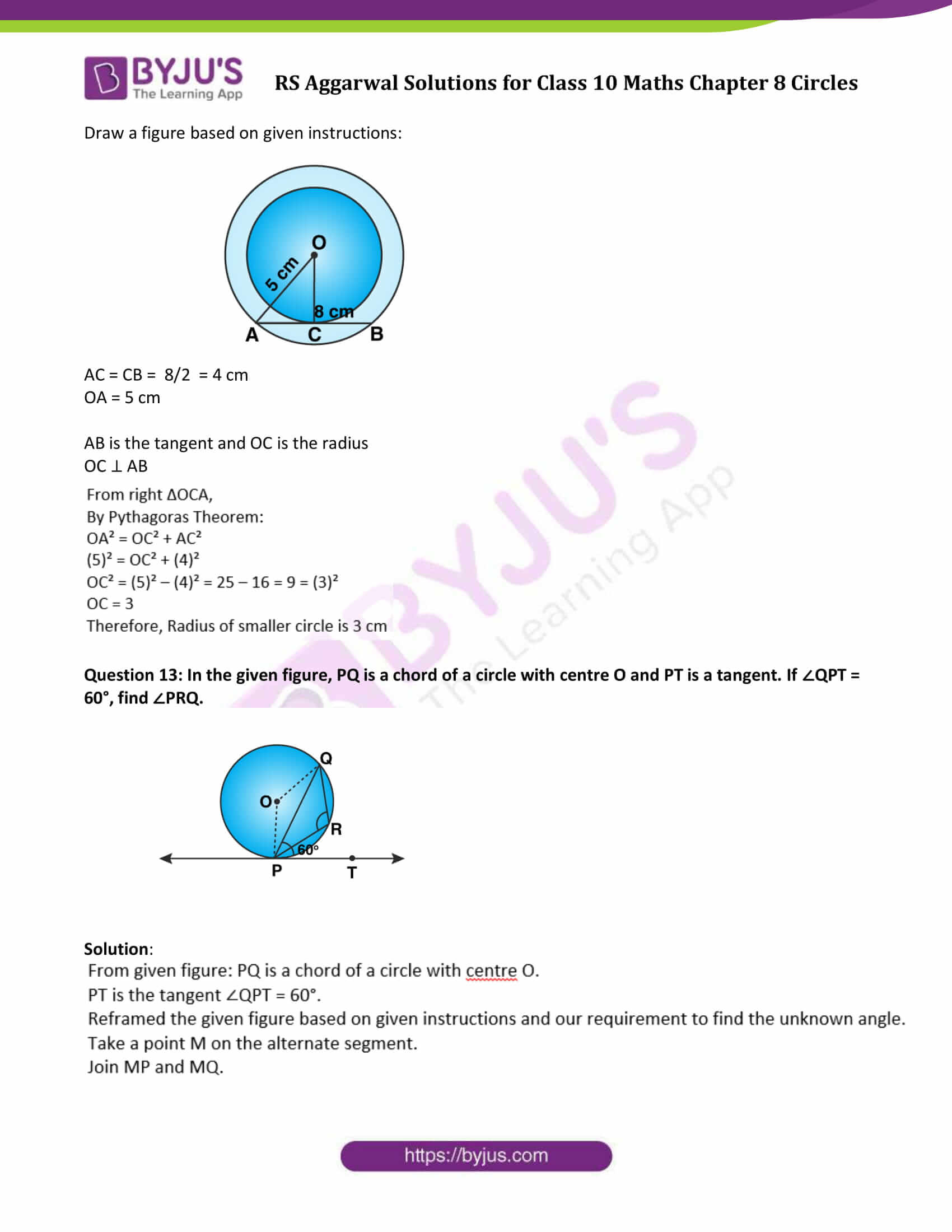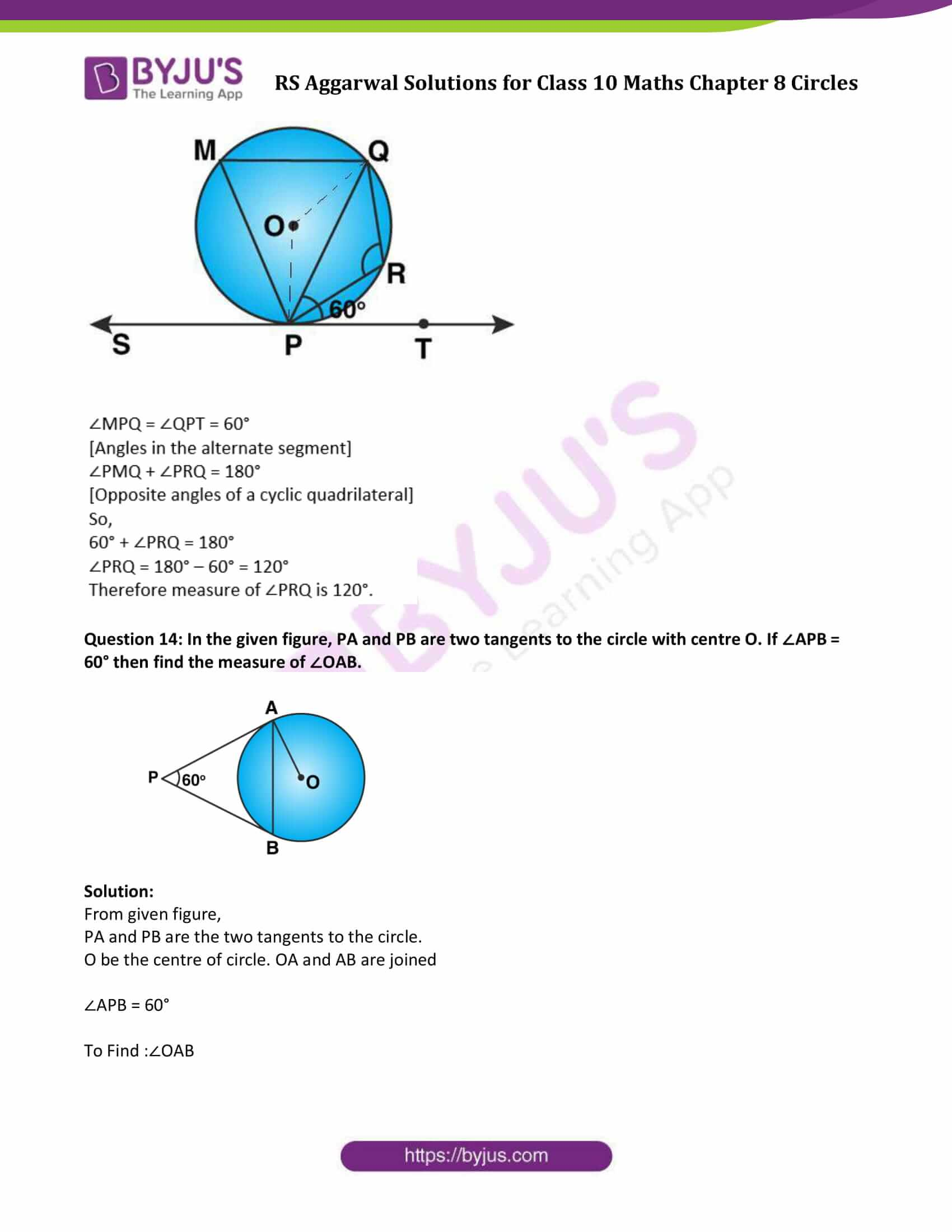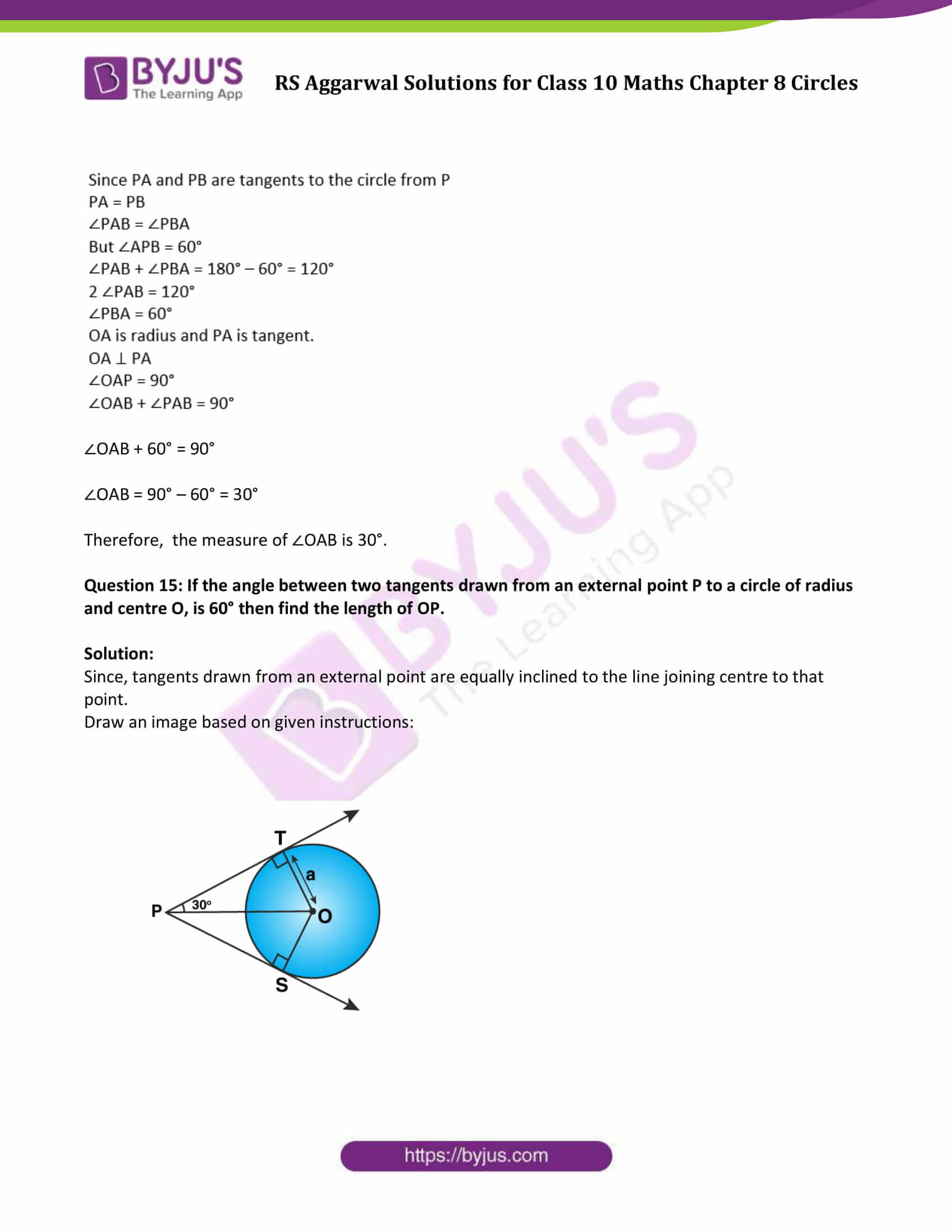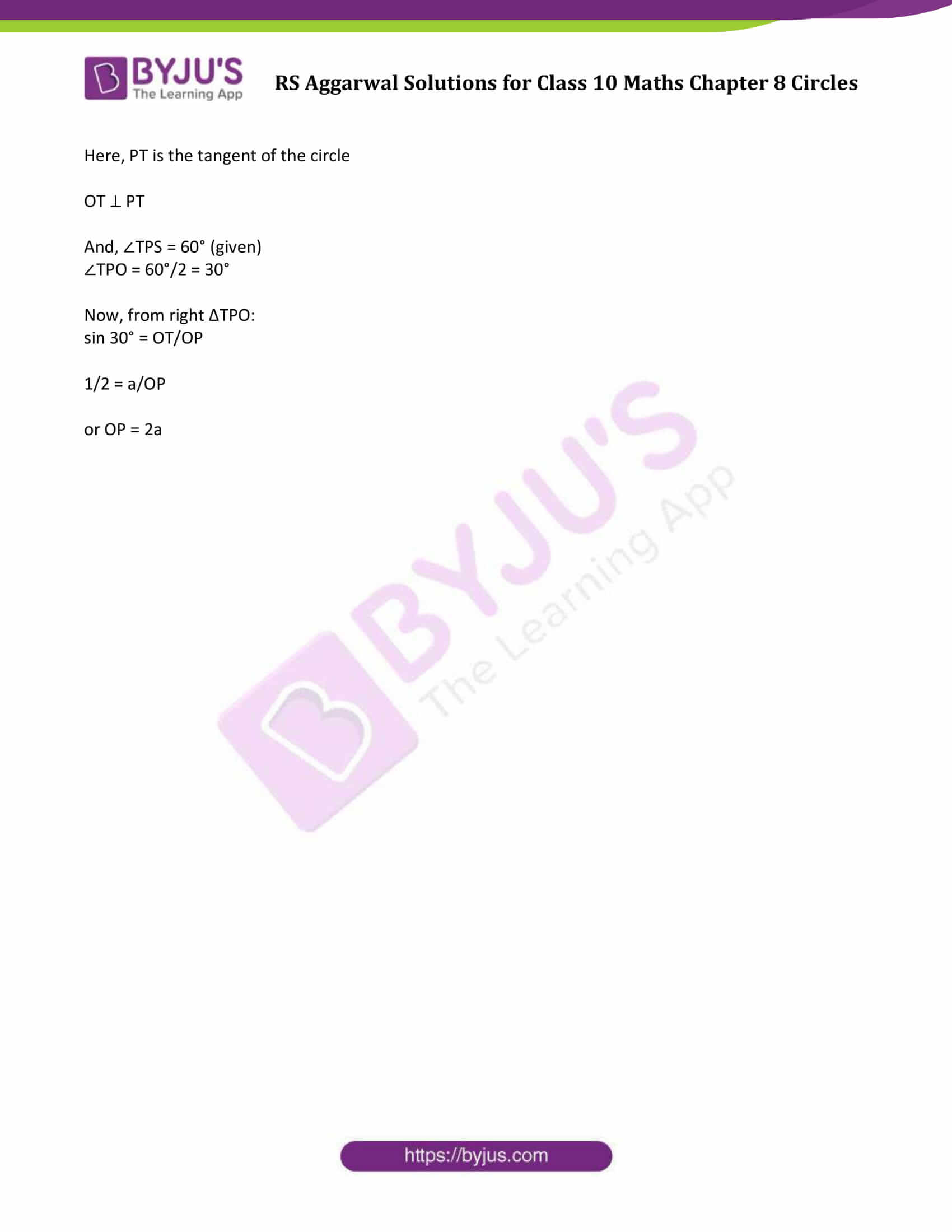### Access other exercise solutions of Class 10 Maths Chapter 8 Circles

Exercise 8A Solutions

### Access Solutions to Maths R S Aggarwal Class 10 Chapter 8 Circles Exercise 8B

Question 1: In the adjoining figure, a circle touches all the four sides of a quadrilateral ABCD whose sides are AB = 6 cm, BC = 9 cm and CD = 8 cm. Find the length of side AD.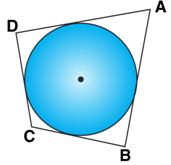Solution:

In the given figure,

A circle touches all the four sides of a quadrilateral ABCD whose sides are AB = 6 cm, BC = 9 cm and CD = 8 cm

Let P, Q, R and S are points where circle touches the sides AB, BC, CD and DA respectively.

Therefore,

AB + CD = BC + AD

6 + 8 = 9 + AD

AD = 14 – 9 = 5

Length of AD is 5 cm.

Question 2: In the given figure, PA and PB are two tangents to the circle with centre O. If ∠APB = 50° then what is the measure of ∠OAB.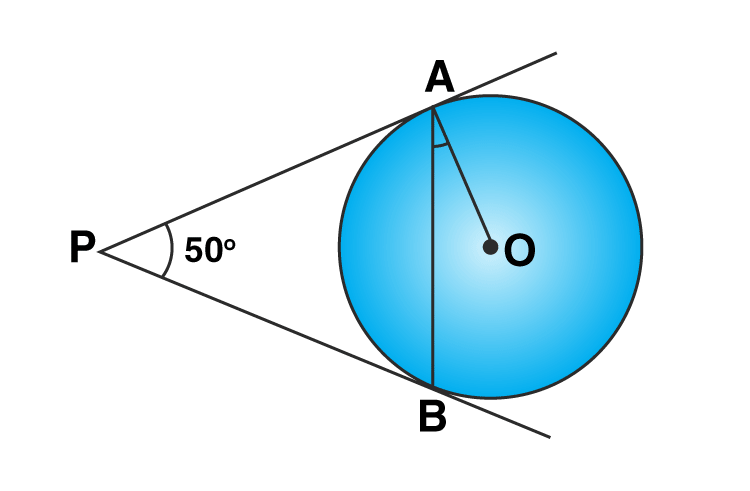Solution:

In the given figure,

∠APB = 50°

PA and PB are two tangents to the circle with centre O.

Join OB.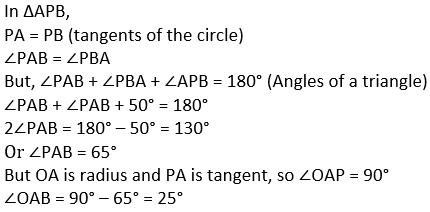Question 3: In the given figure, O is the centre of a circle. PT and PQ are tangents to the circle from an external point P. If ∠TPQ = 70°, find ∠TRQ.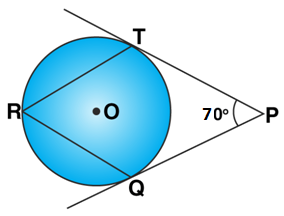Solution:

Here, O is the centre of circle.

PQ and PT are tangents to the circle from a point P

R is any point on the circle. RT and RQ are joined.

∠TPQ = 70°

Now,

Join TO and QO.

∠TOQ = 180° – 70° = 110°

Here, OQ and OT are perpendicular on QP and TP.

∠TOQ is on the centre and ∠TRQ is on the rest part.

∠TRQ = 1/2∠TOQ = ½( 110°) = 55°

Therefore, ∠TRQ = 55 degrees.

Question 4: In the given figure, common tangents AB and CD to the two circles with centres O1 and O2 intersect at E. Prove that AB = CD.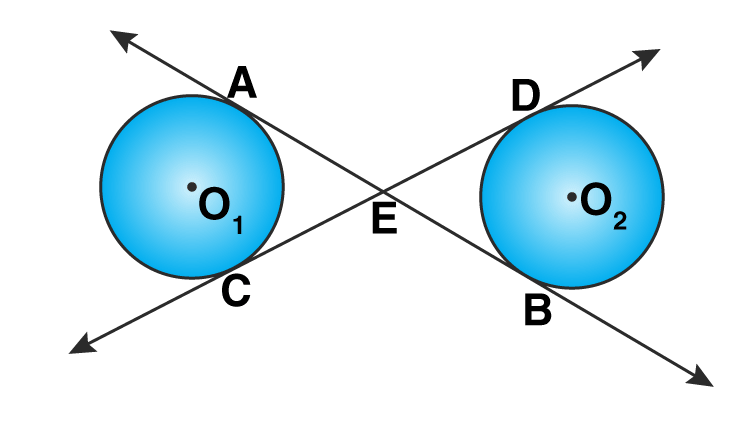Solution:

In the given figure, common tangents

AB and CD are common tangents to the two circles with centres O1 and O2 intersecting at point E.

Circle O1:

EA and EC are tangents

EA = EC …(1)

Circle O2:

EB and ED are tangents

EB = ED …(2)

EA + EB = EC + ED

AB = CD

Hence proved.

Question 5: If PT is a tangent to a circle with centre O and PQ is a chord of the circle such that ∠QPT = 70°, then find the measure of ∠POQ.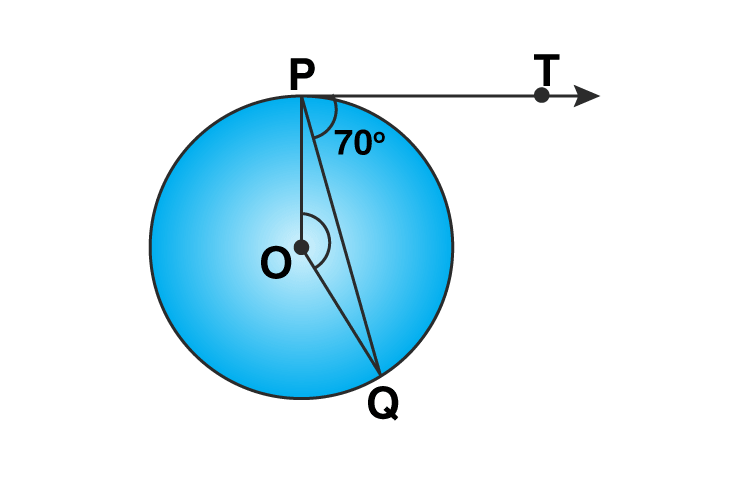Solution: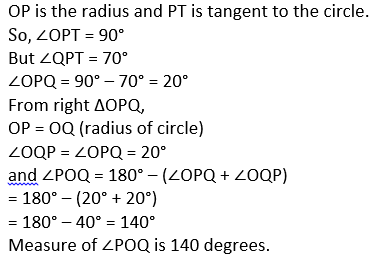Question 6: In the given figure, a triangle ABC is drawn to circumscribe a circle of radius 2 cm such that the segments BD and DC into which BC is divided by the point of contact D, are of lengths 4 cm and 3 cm respectively. If the area of ∆ABC = 21 cm² then find the lengths of sides AB and AC.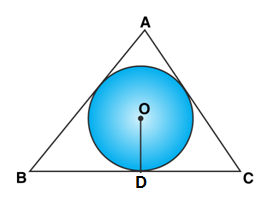Solution:

Given:

∆ABC is circumscribed a circle with centre O and radius 2 cm.

Point D divides BC as

BD = 4 cm, DC = 3 cm, OD = 2 cm

Area of ∆ABC = 21 cm²

Join OA, OB, OC, OE and OF.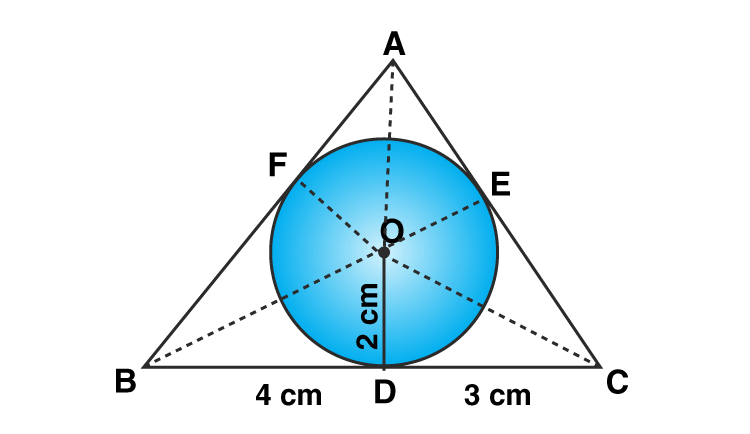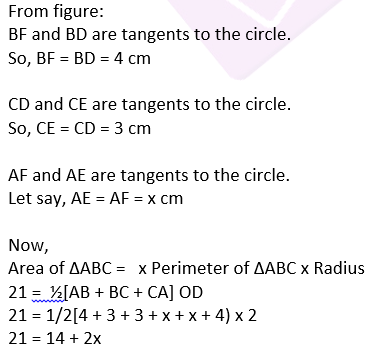x = 3.5

Therefore,

AB = AF + FB = 3.5 + 4 = 7.5 cm

AC = AE + CE = 3.5 + 3 = 6.5 cm

Question 7: Two concentric circles are of radii 5 cm and 3 cm. Find the length of the chord of the larger circle (in cm) which touches the smaller circle.

Solution:

Two concentric circles are of radii 5 cm and 3 cm.

Let O be the centre of circle.

Let AB is chord of larger circle which touches the smaller circle at C.

Join OA and OC.

Below is the figure: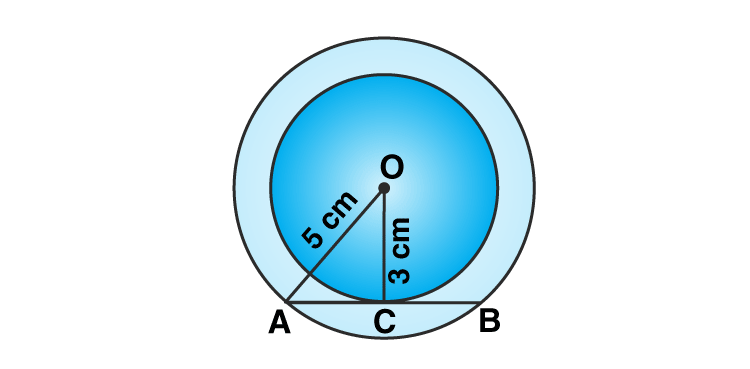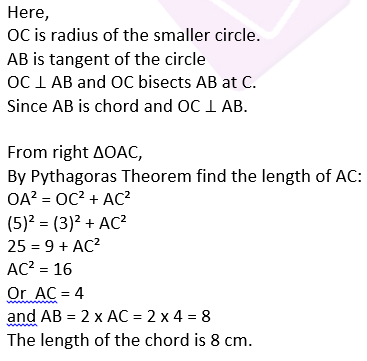Question 8: Prove that the perpendicular at the point of contact of the tangent to a circle passes through the centre.

Solution:

AB is the tangent to the circle at point P

Let O be the centre.

PL ⊥ AB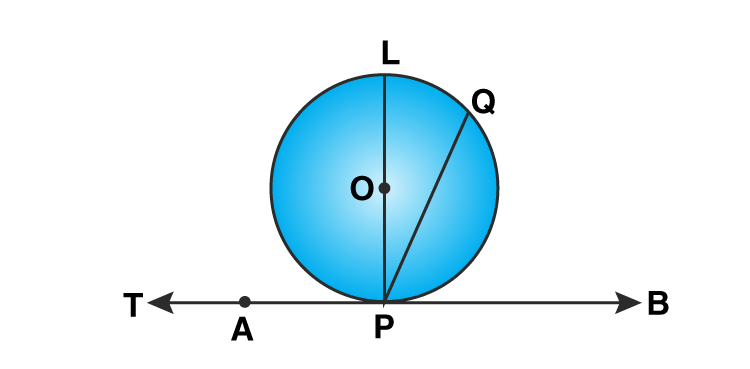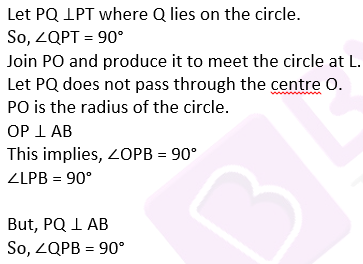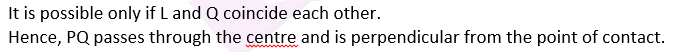Question 9: In the given figure, two tangents RQ and RP are drawn from an external point R to the circle with centre O. If ∠PRQ = 120°, then prove that OR = PR + RQ.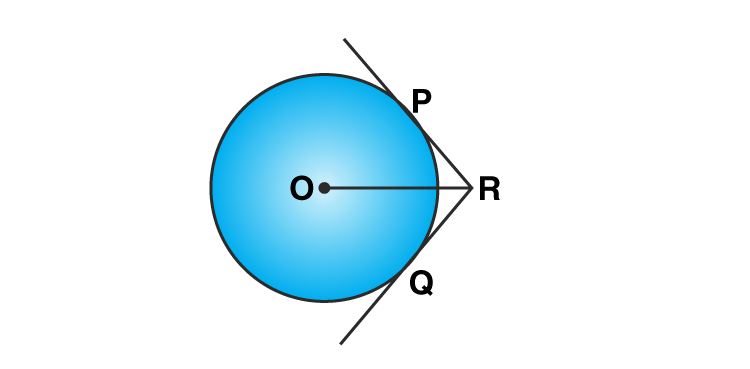Solution: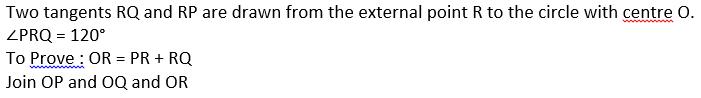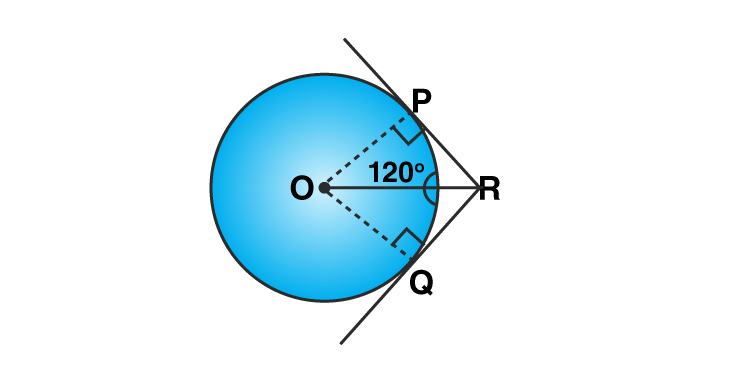∠PRQ = ∠QRO = 120°/2 = 60°

RQ and RP are the tangent to the circle.

OQ ⊥ QR and OP ⊥ PR

Form right ∆OPR,

∠POR = 180° – (90° + 60°) = 30°

and∠QOR = 30°

cos a = PR/OR (suppose ‘a’ be the angle)

cos 60° =PR/OR

1/2 = PR/OR

OR = 2 PR

Again from right ∆OQR,

OR = 2 QR

From both the results, we have

2 PR + 2 QR = 2OR

or OR = PR + RQ

Hence Proved.

Question 10: In the given figure, a circle inscribed in a triangle ABC touches the sides AB, BC and CA at points D, E and F respectively. If AB = 14 cm, BC = 8 cm and CA = 12 cm. Find the lengths AD, BE and CF.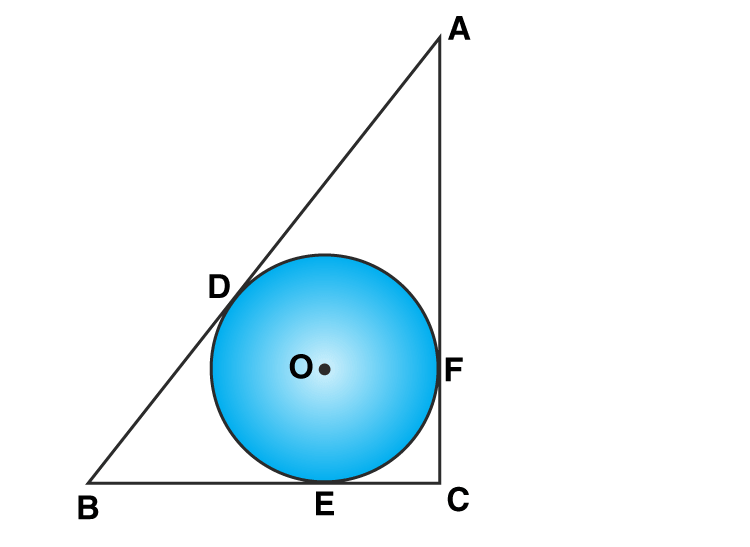Solution:

From given statements, let us reframed the given figure: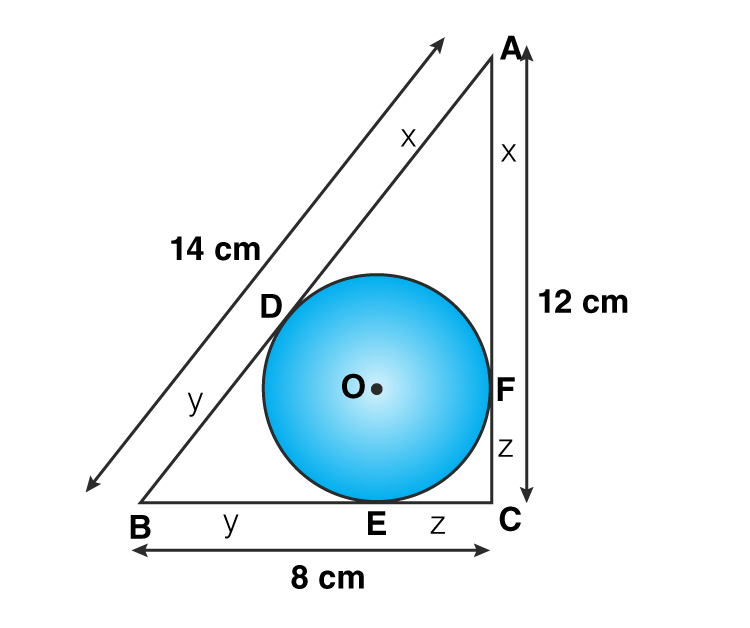AB = 14 cm, BC = 8 cm and CA = 12 cm.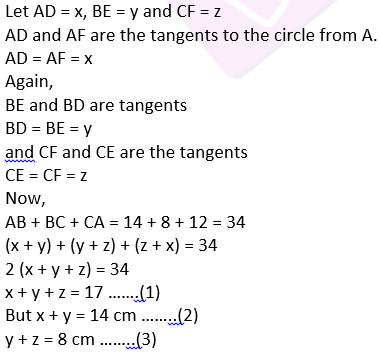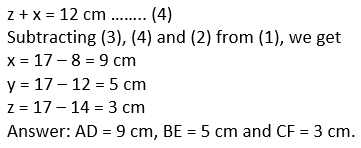Question 11: In the given figure, O is the centre of the circle. PA and PB are tangents. Show that AOBP is a cyclic quadrilateral.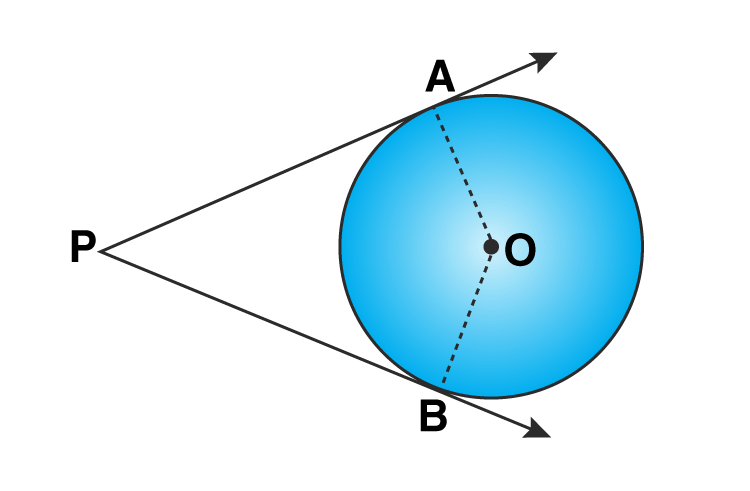Solution: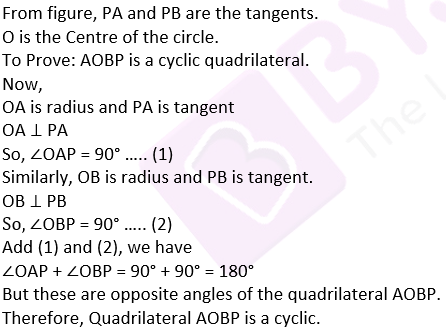Question 12: In two concentric circles, a chord of length 8 cm of the larger circle touches the smaller circle. If the radius of the larger circle is 5 cm then find the radius of the smaller circle.

Solution:

Draw a figure based on given instructions: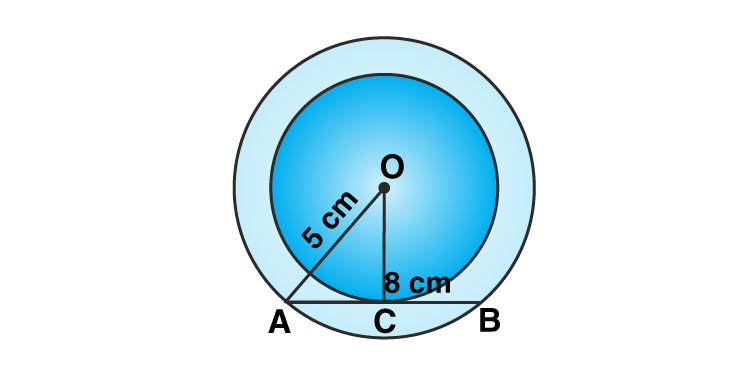AC = CB = 8/2 = 4 cm

OA = 5 cm

AB is the tangent and OC is the radius

OC ⊥ AB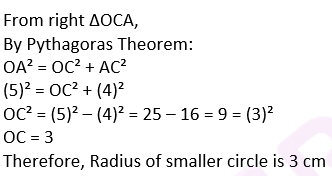Question 13: In the given figure, PQ is a chord of a circle with centre O and PT is a tangent. If ∠QPT = 60°, find ∠PRQ.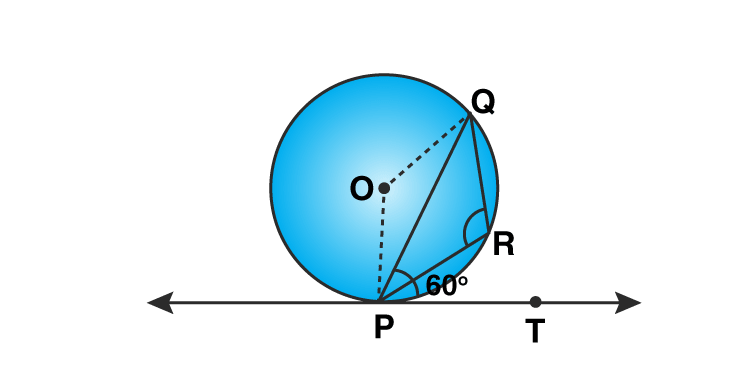Solution: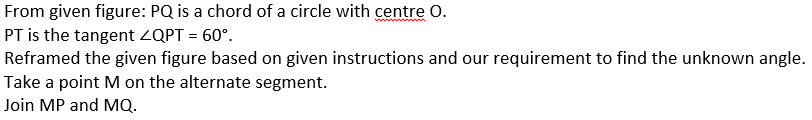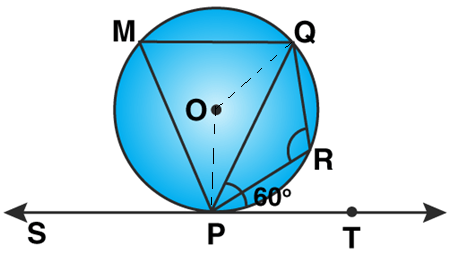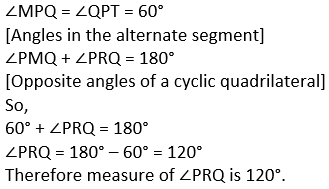Question 14: In the given figure, PA and PB are two tangents to the circle with centre O. If ∠APB = 60° then find the measure of ∠OAB.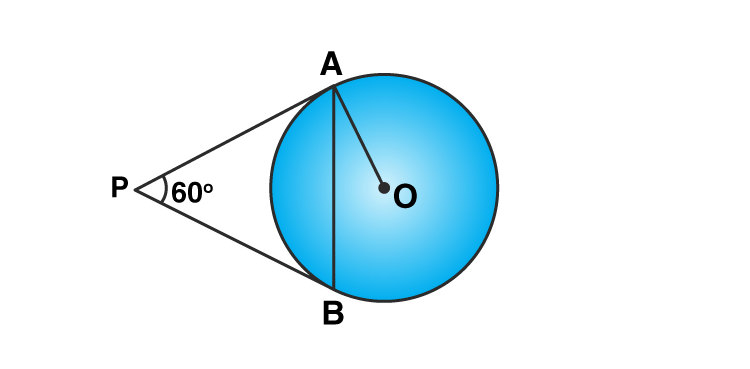Solution:

From given figure,

PA and PB are the two tangents to the circle.

O be the centre of circle. OA and AB are joined

∠APB = 60°

To Find :∠OAB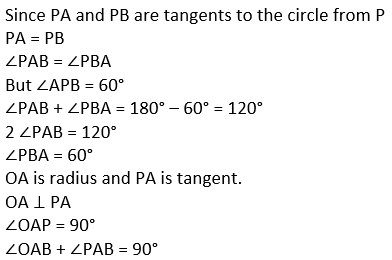∠OAB + 60° = 90°

∠OAB = 90° – 60° = 30°

Therefore, the measure of ∠OAB is 30°.

Question 15: If the angle between two tangents drawn from an external point P to a circle of radius and centre O, is 60° then find the length of OP.

Solution:

Since, tangents drawn from an external point are equally inclined to the line joining centre to that point.

Draw an image based on given instructions: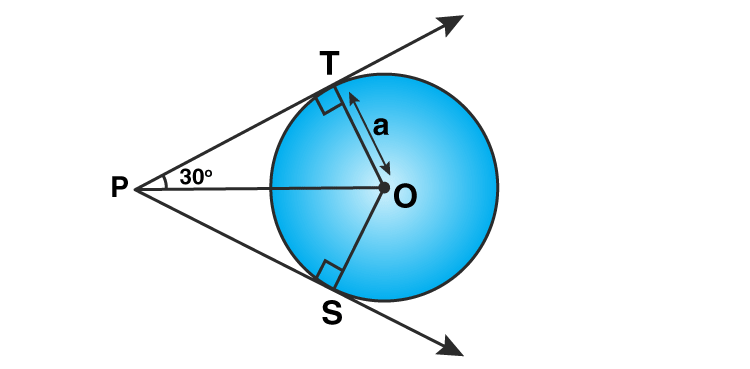Here, PT is the tangent of the circle

OT ⊥ PT

And, ∠TPS = 60° (given)

∠TPO = 60°/2 = 30°

Now, from right ∆TPO:

sin 30° = OT/OP

1/2 = a/OP

or OP = 2a

## R S Aggarwal Solutions for Class 10 Maths Chapter 8 Circles Exercise 8B

Class 10 Maths Chapter 8 Circles Exercise 8B is based on important theorems on circle tangents. Some of the important theorems are:

-The tangent at any point of a circle is perpendicular to the radius through the point of contact.

-Converse of the tangent theorem: A line drawn through the end of a radius and perpendicular to it is a tangent to the circle.

-The lengths of tangents drawn from an external point to a circle are equal.